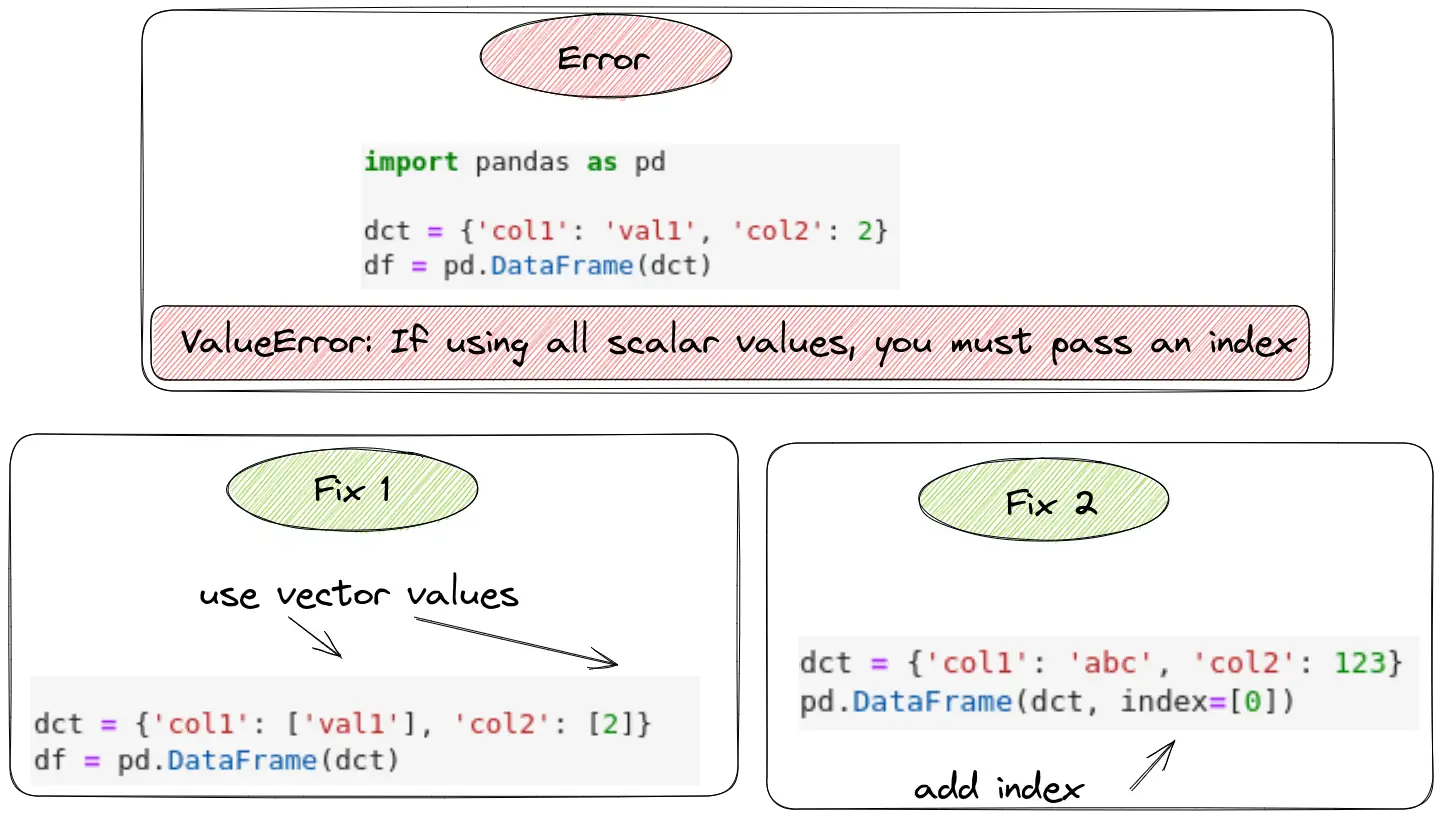In this tutorial, we'll take a closer look at the Pandas error:

"ValueError: If using all scalar values, you must pass an index"

You can find explanation and solution on the image below:Quick fixes:

``````pd.DataFrame(dct, index=)
``````

(2) use vector values

``````dct = {k:[v] for k,v in dct.items()}
``````

(3) Wrap with list

``````dct = {'col1': ['val1'], 'col2': }
pd.DataFrame(dct, index=)
``````

First, we'll create an example of how to produce it. Next, we'll explain the leading cause of the error. And finally, we'll see how to fix it.

## Example

Now, let's see an example that generates a Pandas error:

ValueError: If using all scalar values, you must pass an index

``````import pandas as pd

dct = {'col1': 'val1', 'col2': 2}
df = pd.DataFrame(dct)
``````

So we get the error above:

``````ValueError: If using all scalar values, you must pass an index
``````

## Cause

The error is raised because we pass only scalar values. What is a scalar value?

Definition
Scalar value is a single value.

So the reason is that we are using a dictionary with single values.

``````{
"col1": "val1",
"col2": 2
}
``````

So using single values rather than lists or arrays will raise the error: `ValueError: If using all scalar values, you must pass an index`

## Solution - use vector values

So one solution is to use vector values instead of scalar values. In practice this means turning:

``````{
"col1": "val1",
"col2": 2
}
``````

to

``````{
"col1": ["val1"],
"col2": 
}
``````

This would resolve the error. We can use dict comprehensions in order to workaround the error like - `{k:[v] for k,v in dct.items()}`:

``````dct = {'col1': 'abc', 'col2': 123}
dct = {k:[v] for k,v in dct.items()}
pd.DataFrame(dct)
``````

This will solve the error and create DataFrame like:

col1 col2
0 abc 123

## Solution - wrap with list

Another solution is to wrap the whole dictionary with list:

``````dct = [{'col1': 'abc', 'col2': 123}]

df = pd.DataFrame(dct)
``````

The code above will create DataFrame without error.

col1 col2
0 abc 123

or:

``````dct = {'col1': ['abc'], 'col2': }

df = pd.DataFrame(dct)
``````

which has the same output in this case:

col1 col2
0 abc 123

If we don't pass an index, it will raise an error that the 'DataFrame()' constructor requires an index to be passed.

So the final solution is to add a index in order to solve:

``````ValueError: If using all scalar values, you must pass an index
``````
``````dct = {'col1': 'abc', 'col2': 123}

pd.DataFrame(dct, index=)
``````

## Conclusion

We've explained Pandas error "ValueError: If using all scalar values, you must pass an index".

Then, we discussed how to produce the error and the cause of the problem. Lastly, we discussed several solutions to resolve the error.

Article starts with nice visualization and quick fixes for the error.